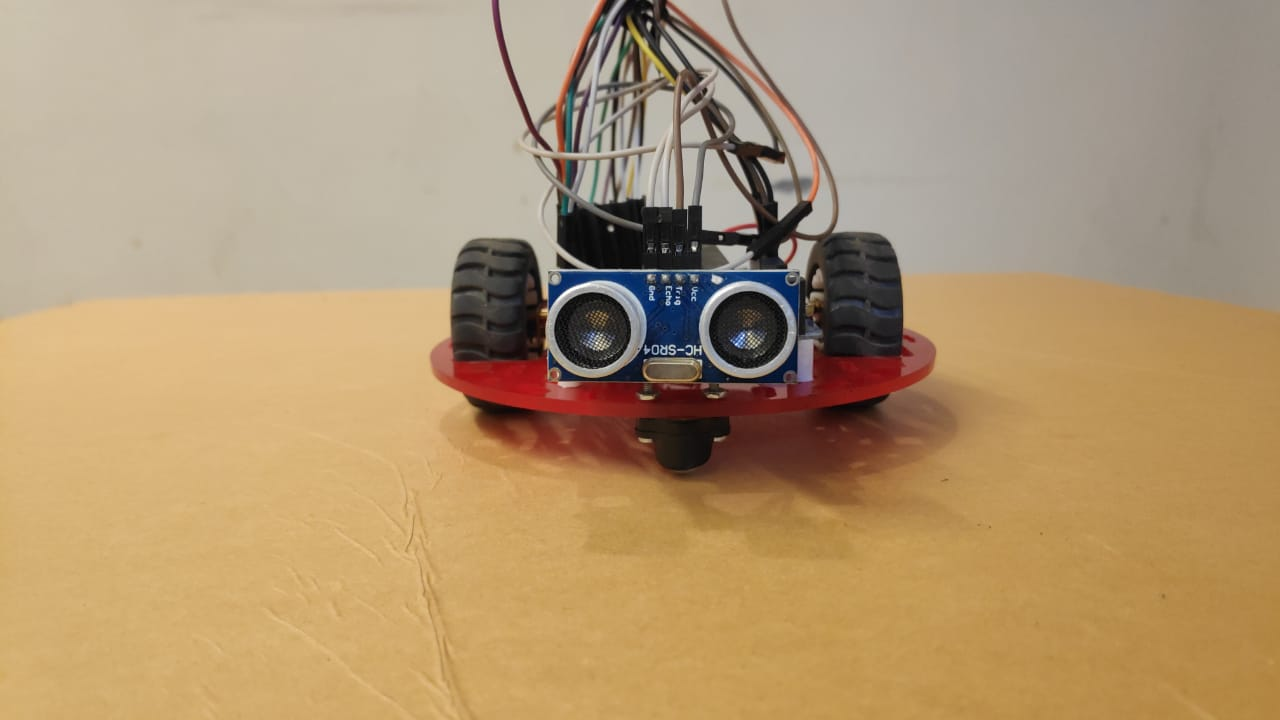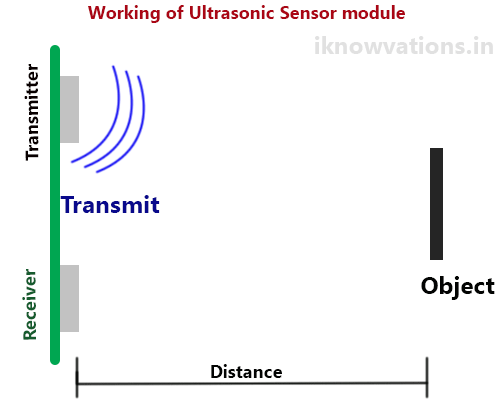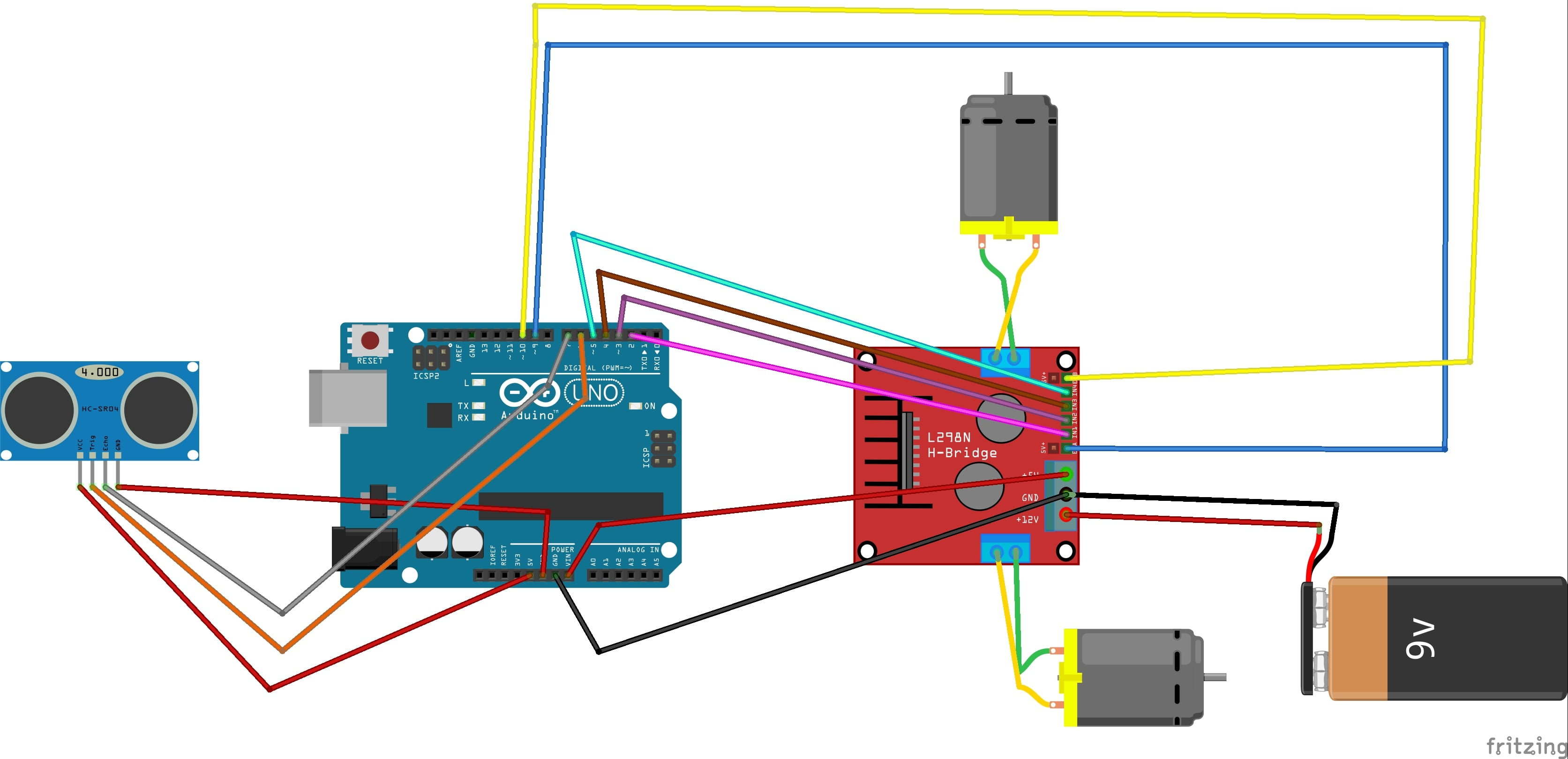Arduino This is a fork of Obstacle avoiding robot

# Arduino-based Smart RobotApril 02, 2020 by Neha Das
ShareLearn an easy way to create an obstacle avoiding robot using Arduino.

## Hardware

 1 L293D 2 wheels 20 jumper wires 1 arduino uno 1 ultrasonic sensor

## Software

 1 arduino ide
This is a very simple and effective project for beginners. An obstacle avoiding robot is a smart robot that avoids obstacles in its path. You can also make a maze solving robot using it. Here I am sharing code and connections.

## Working Principle

Here I am using an ultrasonic sensor to measure distance. The sensor produce an ultrasonic sound wave, That wave is reflected back from any solid object. The speed of sound is 340 meters per second. we measure the time and apply a simple formula (Distance= speed * time) we get the distance and divide this distance with 2 will give you the exact distance.

You can program your robot according to this distance the same thing I did in my code. When the distance is less than 15cm I instruct my robot to take left. if distance id greater then 15 go straight.``````#define trigPin 6
#define echoPin 7
#define left_m1 2
#define left_m2 3
#define rgt_m1 4
#define rgt_m2 5
#define led 13
void setup()
{ Serial.begin(9600);
pinMode(trigPin, OUTPUT);
pinMode(echoPin, INPUT);
pinMode(left_m1, OUTPUT);
pinMode(left_m2, OUTPUT);
pinMode(rgt_m1, OUTPUT);
pinMode(rgt_m2, OUTPUT);
pinMode(led, OUTPUT);
pinMode(9, OUTPUT);
pinMode(10, OUTPUT);
}
void forward()
{
analogWrite(5, 30);
analogWrite(6, 30);
digitalWrite(left_m1, LOW);
digitalWrite(left_m2, HIGH);
digitalWrite(rgt_m1, LOW);
digitalWrite(rgt_m2, HIGH);
digitalWrite(led, HIGH);
}
void back()
{
analogWrite(5, 30);
analogWrite(6, 30);
digitalWrite(left_m1, HIGH);
digitalWrite(left_m2, LOW);
digitalWrite(rgt_m1, HIGH);
digitalWrite(rgt_m2, LOW);
digitalWrite(led, HIGH);
}
void right()
{
analogWrite(5, 30);
analogWrite(6, 30);
digitalWrite(left_m1, HIGH);
digitalWrite(left_m2, LOW);
digitalWrite(rgt_m1, LOW);
digitalWrite(rgt_m2, HIGH);
digitalWrite(led, HIGH);
}
void left()
{
analogWrite(5, 30);
analogWrite(6, 30);
digitalWrite(left_m1, LOW);
digitalWrite(left_m2, HIGH);
digitalWrite(rgt_m1, HIGH);
digitalWrite(rgt_m2, LOW);
digitalWrite(led, HIGH);
}
void stops()
{
digitalWrite(left_m1, LOW);
digitalWrite(left_m2, LOW);
digitalWrite(rgt_m1, LOW);
digitalWrite(rgt_m2, LOW);
digitalWrite(led, LOW);
}
void loop()
{
long distance, duration;
digitalWrite(trigPin, LOW);
delayMicroseconds(2);
digitalWrite(trigPin, HIGH);
delayMicroseconds(10);
digitalWrite(trigPin, LOW);

duration = pulseIn(echoPin, HIGH);
distance = (duration / 2) / 29.1;
Serial.println(distance);
if (distance >= 25 )
{
forward();
Serial.print("f");
}
else if (distance <= 24)
{

left();

}

delay(400);

}``````Assemble your robot according to above schismatics

Ultrasonic ----------- Arduino------------ motor driver

Eco                                      7

Trig                                      6

2                                  IN 1

3                                  IN2

4                                  IN3

5                                  IN4